## Newton's Laws Applications Review

#### Navigate to:

Review Session Home - Topic Listing

Forces in 2 Dimensions - Home || Printable Version || Questions with Links

Answers to Questions:  All || #1-12 || #13-21 || #22-30

 [ #1 | #2 | #3 | #4 | #5 | #6 | #7 | #8 | #9 | #10 | #11 | #12  ]

### Part A: Drawing Free-Body Diagrams

1. Construct free-body diagrams for the following physical situations.

 a. A ball is dropped from rest from the top of a building. Assume negligible air resistance.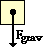In the absence of Fair, the only force acting upon the ball is gravity. It is a projectile. b. After being thrown, a football is moving upwards and rightwards towards the peak of its trajectory. Assume negligible air resistance.In the absence of Fair, the only force acting upon the ball is gravity. It is a projectile. Note that an upwards moving object does not need an upwards force. Only an upwards accelerating object requires an upwards force. c. After reaching a terminal velocity, a falling skydiver then opens up the parachute.When the terminal velocity was reached, Fair = Fgrav. Then the parachute was opened, making Fair even greater than before. This is represented by the larger arrow. d. An air track glider is gliding to the right at constant velocity.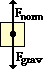There is no rightwards force. A rightwards force would only be required if there is a rightwards acceleration. If the glider is gliding; there is no mention of it being pushed or pulled (Fapp) and if there is a constant velocity, there must be balanced forces. e. A car is skidding to a stop while travling to the right.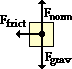There is no rightwards force. A rightwards force would only be required if there is a rightwards acceleration. If the car is skidding (wheels are locked), friction acts in a direction opposite its motion to slow it down. f. A downward moving elevator (held by a cable) slows down.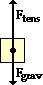The cable supplies the tension force. Since the elevator is moving downwards and slowing down, there must be more upwards force than the downwards gravity force. g. A 25.0-N force is applied at a 30-degree angle to a crate in order to accelerate it rightward across a rough, horizontal surface.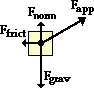The applied force is upwards and rightwards. Its upward component contributes to the upwards Fnorm to balance the force of gravity. (Note the relative size of Fnorm.) h. A picture hangs summetrically by two wires oriented at angles to the vertical.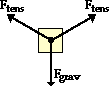The force exerted by a wire is a tension force. With two wires, there would be two upwards-pulling tension forces. The down force is gravity. i. A large crate slowly accelerated down a steep and rough inclined plane.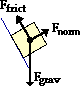There are only three forces present. The Fpar and Fperp are merely components of gravity; they are not separate forces. The normal force is perpendicular to the surface (drawn in blue).

 Useful Web Links Types of Forces || Drawing Free-Body Diagrams

### Part B: Conceptual Notions of Force and Motion

Express your understanding of concepts of force, acceleration, and motion by answering the following questions.

2. Which of the following are always true of an object that is at equilibrium? Include all that apply.

1. All the forces acting upon the object are equal.
2. The object is at rest.
3. The object is moving and moving with a constant velocity.
4. The object has an acceleration of zero.
5. There is no change in the object's velocity.
6. The sum of all the forces is 0 N.
7. All the forces acting upon an object are balanced.
 Answer: DEFG These (DEFG) statements are always true. Statements ABC might be true but are not always true.

 Useful Web Links Equilibrium and Statics || The Big Misconception

[ #1 | #2 | #3 | #4 | #5 | #6 | #7 | #8 | #9 | #10 | #11 | #12  ]

3. Which of the following are never true of an object that is at equilibrium? Include all that apply.

1. The object is accelerating.
2. The object is at rest.
3. The object is moving in a circle at constant speed.
4. All the forces acting upon the object are equal.
5. The object is in free-fall.
6. The object is falling and has reached its terminal velocity.
7. There is a net force acting upon the object.
8. The object is moving and moving with a constant velocity.
 Answer: ACEG An object that is at equilibrium can never be accelerating; its acceleration MUST be 0 m/s/s. Thus, A is an answer; and because an object in free-fall (E) and an object moving in a circle (C) are also accelerating, they must be counted as answers as well. (NOTE: an object moving in a circle is changing its direction and as such has an acceleration.) If there is a net force (G), then by definition the object is not at equilibrium. Choices B, D, F and H could be true of an object at equilibrium (though none of them are always true).

 Useful Web Links Equilibrium and Statics || The Big Misconception

[ #1 | #2 | #3 | #4 | #5 | #6 | #7 | #8 | #9 | #10 | #11 | #12  ]

4. Big Bubba has a mass of 100 kg on the earth. What is Big Bubba's mass on the moon where the force of gravity is approximately 1/6-th that of Earth's? ________ Explain or show your work.

 Answer: 100 kg Mass is the amount of matter present in the object and is independent of the weight of the object. The weight or force of gravity acting upon an object depends upon the mass and the acceleration of gravity (Fgrav = m•g). Because g is different on different planets and locations in the universe, the force of gravity or weight of an object will not always be the same. If Big Bubba goes to the moon, he will weigh less due to the decreased acceleration of gravity. However, he will still look the same, still have the same amount of matter, and still have a mass of 100 kg. KNOW the distinction between mass and weight.

 Useful Web Links Mass vs. Weight vs. Force of Gravity

[ #1 | #2 | #3 | #4 | #5 | #6 | #7 | #8 | #9 | #10 | #11 | #12  ]

5. Little Billie weight 360 N on Earth. What is Little Billie's mass on the moon where the force of gravity is approximately 1/6-th that of Earth's? ________ Explain or show your work.

 Answer: 37 kg (approx.) The acceleration of gravity on the moon is 1/6-th the value of earth's; this explains why the force of gravity is 1/6-th that of earth's. The force of gravity (Fgrav) on the moon is calculated using the equation Fgrav = m•g where g =1.6 m/s/s (on the moon). If Little Billie's weight on the moon is 1/6-th of 360 N, then Little Billie weighs 60 N on the moon. Inserting 60 N into the equation with 1.6 m/s/s as the value of g, Little Billie's mass can be calculated to be approximately 37 N. (Alternatively, simply find Billie's mass on earth from his weight of 360 N and then conclude that this mass value is not going to change when he goes to the moon.)

 Useful Web Links Mass vs. Weight vs. Force of Gravity

[ #1 | #2 | #3 | #4 | #5 | #6 | #7 | #8 | #9 | #10 | #11 | #12  ]

6. TRUE or FALSE:

An object which is moving rightward has a rightward force acting upon it.
 Answer: FALSE A rightward acceleration would require a rightward net force. But if an object is moving rightward and slowing down (a leftwards acceleration), then there is certainly not a rightward net force and possibly not even a rightward force at all. For instance, if a car is moving rightward and skidding to a stop (with wheels locked), then there is no rightward force upon the car. The only horizontal force is a leftward force of friction which serves to slow the car down. (If you're getting stuck on this question and ones like them, it might be time to read the page titled "The Big Misconceptsion"; use the link below.)

 Useful Web Links The Big Misconception

[ #1 | #2 | #3 | #4 | #5 | #6 | #7 | #8 | #9 | #10 | #11 | #12  ]

7. How much net force is required to keep a 5-kg object moving rightward with a constant velocity of 2 m/s? ________ Explain or show your work.

 Answer: 0 N If the velocity is constant, then the acceleration is 0 m/s/s and the net force is zero. A net force is only required in order to accelerate an object. Yes, this means that an object can be moving to the right and NOT have a rightward's net force. (If you're getting stuck on this question and ones like them, it might be time to read the page titled "The Big Misconceptsion"; use the link below.)

 Useful Web Links The Big Misconception

[ #1 | #2 | #3 | #4 | #5 | #6 | #7 | #8 | #9 | #10 | #11 | #12  ]

8. TRUE or FALSE:

For an object resting upon a non-accelerating surface, the normal force is equal to the force of gravity.
 Answer: FALSE In this instance, the normal force could be equal to the force of gravity. But all that we can conclusively know is that the all the vertical forces sum up to 0 N. If the object is upon an incline, then the normal force will not be equal to the force of gravity. Or if there is another force with an upward or downward component, then the normal force is not equal to the force of gravity.

[ #1 | #2 | #3 | #4 | #5 | #6 | #7 | #8 | #9 | #10 | #11 | #12  ]

9. An object rests upon an inclined plane. If the angle of incline is increased, then the normal force would _______.

 a. increase b. decrease c. remain the same
 Answer: B The normal force is equal to the perpendicular component of the weight vector. So Fnorm = Fperp = m•g•cos(angle). If the incline angle is increased, the cos(angle) decreases towards 0; thus, the normal force decreases as well. Of course, the extreme is when the angle is 90 degrees and there is no normal force.

[ #1 | #2 | #3 | #4 | #5 | #6 | #7 | #8 | #9 | #10 | #11 | #12  ]

10. Three pictures of equal weight (20 N) are hung by wires in three different orientations. In which orientation are the wires least likely to break? _______ Explain why.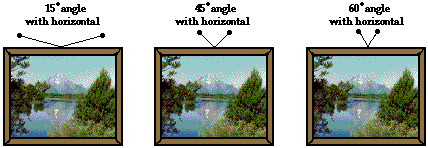Answer: 60-degree angle to the horizontal The tension in the wire will have the greatest impact upon its tendency to break. As the wire becomes most vertically oriented, the horizontal component of the tension force is reduced and the tension becomes less. The moral of the story -- to support the weight of a picture, one only needs to pull upwards, not leftwards and rightwards. Of course, the best arrangement would be two wires pulling completely vertically.

 Useful Web Links Equilibrium and Statics

[ #1 | #2 | #3 | #4 | #5 | #6 | #7 | #8 | #9 | #10 | #11 | #12  ]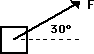11. A 50-N force is applied at an angle of 30 degrees north of east. This would be the same as applying two forces at

1. 43 N, east and 7 N, north
2. 35 N, east and 15 N, north
3. 25 N, east and 25 N, north
4. 43 N, east and 25 N, north
 Answer: D A 50-N force at 30 degrees would have two components - 43 N, east and 25 N, north. These two forces would be equal to the 50-N force at 30 degrees N of E. They are calculated as: Feast = (50 N)*cos(30 deg) Fnorth = (50 N)*sin(30 deg)

 Useful Web Links Resolution of Forces

[ #1 | #2 | #3 | #4 | #5 | #6 | #7 | #8 | #9 | #10 | #11 | #12  ]

12. Which one(s) of the following force diagrams depict an object moving to the right with constant speed? Write all possible answers (if any) in the blank: ________________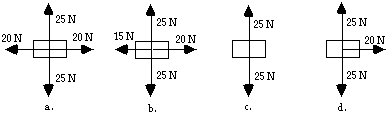Answer: AC Only A and C could have a constant speed. Both B and D would have an acceleration that is directed rightwards. Do not confuse acceleration, motion, and force. A rightwards acceleration requires that there be a rightwards net force, On the other hand, a rightwards motion could have no net force, a leftward net force or a rightwards net force; it depends upon whether the object is slowing down, speeding up or maintaining a constant speed. Only A and C show a balance of forces, so only A and C are moving with constant speed. If you're getting stuck on this question and ones like them, it might be time to read the page titled "The Big Misconception"; use the link below.

 Useful Web Links The Big Misconception

[ #1 | #2 | #3 | #4 | #5 | #6 | #7 | #8 | #9 | #10 | #11 | #12  ]

#### Navigate to:

Review Session Home - Topic Listing

Forces in 2 Dimensions - Home || Printable Version || Questions with Links

Answers to Questions:  All || #1-12 || #13-21 || #22-30

## You Might Also Like ...

Users of The Review Session are often looking for learning resources that provide them with practice and review opportunities that include built-in feedback and instruction. If that is what you're looking for, then you might also like the following:

The Calculator Pad includes physics word problems organized by topic. Each problem is accompanied by a pop-up answer and an audio file that explains the details of how to approach and solve the problem. It's a perfect resource for those wishing to improve their problem-solving skills.

Visit: The Calculator Pad Home | Calculator Pad - Forces in Two Dimensions

2. Minds On Physics the App Series

Minds On Physics the App ("MOP the App") is a series of interactive questioning modules for the student that is serious about improving their conceptual understanding of physics. Each module of the series covers a different topic and is further broken down into sub-topics. A "MOP experience" will provide a learner with challenging questions, feedback, and question-specific help in the context of a game-like environment. It is available for phones, tablets, Chromebooks, and Macintosh computers. It's a perfect resource for those wishing to refine their conceptual reasoning abilities. Part 2 of the series includes Forces in Two Dimensions.

Visit: MOP the App Home || MOP the App - Part 2